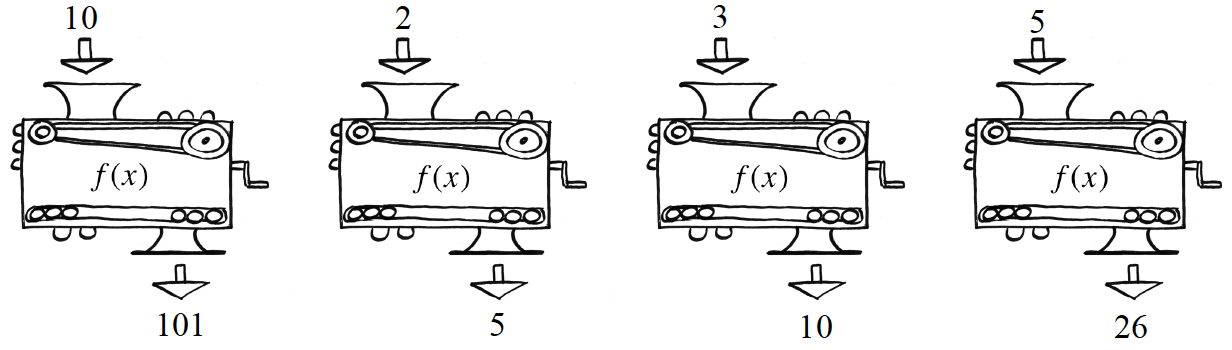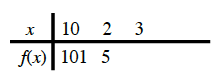### Home > CCA2 > Chapter Ch2 > Lesson 2.2.5 > Problem2-168

2-168.

Remember function machines? Each of the following pictures shows how the same machine changes the given x-value into a corresponding $f (x)$ value. Find the equation for this machine. Homework Help ✎Copy and complete the table for the function.$f(x)=x^2+1$